Home

 Article 40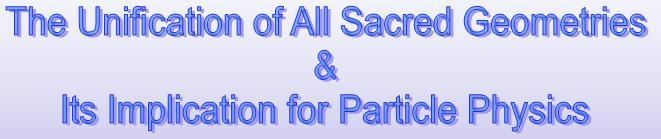Part 4 by Stephen M. Phillips Flat 3, 32 Surrey Road South. Bournemouth. Dorset BH4 9BP. England. Website: http://www.smphillips.mysite.com 1

Figure 1

The graph shows the positions of the 62 vertices of the disdyakis triacontahedron projected onto the XY plane with the Z axis running through two diametrically opposite C vertices. 60 vertices are corners of polygons in 15 layers. Solid lines of the same colour connect vertices at the same height above the XY plane. Dashed lines of the same colour connect vertices at the same depth below the XY plane. Vertices at the same height form either triangles or 6-sided polygons, with six A vertices in the XY plane located at the corners of a hexagon. Suppose that the 60 sectors of these polygons are each constructed from three triangles. The latter share one corner at the centre of each sector. 15 of the corners of the 180 new triangles are the centres of the polygons, so that they have (15+60=75) corners inside the polyhedron and 60 corners that are polyhedral vertices. Including the C vertices at its apex and nadir, there are (75+60+2=137) vertices. This number is one of the most important numbers in physics because it defines approximately as its reciprocal the fine-structure constant e2/ħ c that measures the strength of the coupling of electrically charged particles to the electromagnetic field. Appropriately, the number determining the electron structure of atoms is embodied in the sheets of vertices of the disdyakis triacontahedron as the number of vertices needed to construct its faces and interior from triangles.

If the triangles are now turned into tetractyses, the transformation requires 840 new yods. This is the number of turns in each helical whorl of the heterotic superstring as it spirals 2½ times around its spin axis, making half a complete circuit. The disdyakis triacontahedron embodies this structural parameter of the heterotic superstring.

2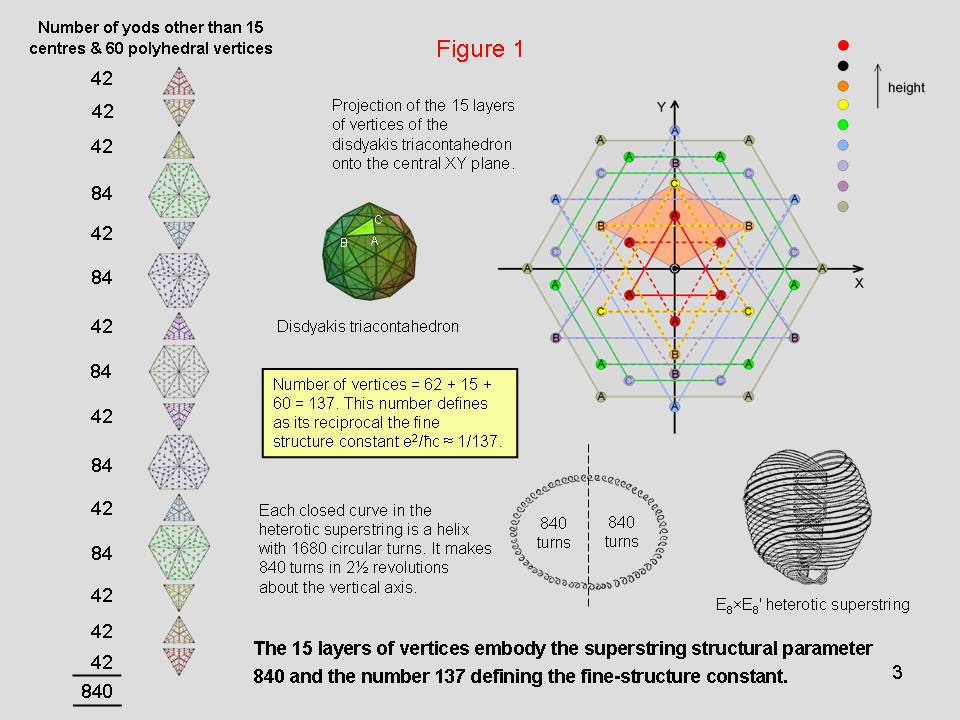Figure 2

Suppose that the seven polygons above the XY plane formed by vertices of the disdyakis triacontahedron are divided into their sectors and the latter then constructed from three tetractyses. There are 378 yods other than polyhedral vertices surrounding the centres of the seven polygons. This is the number of yods needed to turn the 42 triangles of the 3-dimensional Sri Yantra into tetractyses. Their 42 black centres correspond to the 42 black yods surrounding the centre of the uppermost triangle that are not vertices of the polyhedron. The 84 red yods at corners of tetractyses correspond to the 84 similar red yods in the next two triangles. The 84 green, hexagonal yods on the 42 edges of the 14 tetractyses in the fourth layer of the Sri Yantra correspond to the 84 green yods in the other two triangles. The 168 yellow, hexagonal yods on the 84 edges of the 28 triangles in the first three layers correspond to the 168 yellow yods in the pair of six-sided polygons.

In either case, the 378 yods symbolize the 378 rising and falling intervals other than the octave between the notes of the seven musical scales.

4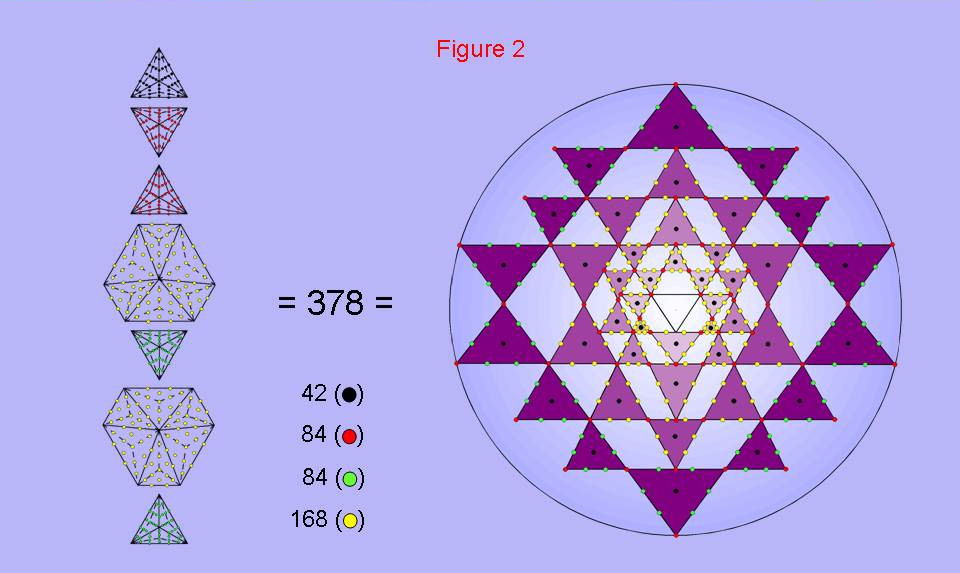There are 378 yods other than polyhedral vertices surrounding the centres of the seven polygons               above the equatorial plane of the disdyakis triacontahedron. This is the number of yods in the               42 triangles of the 3-d Sri Yantra. They symbolize the 378 rising and falling intervals below the               octave of the notes in the seven musical scales. 5

Figure 3

The I Ching table, the inner Tree of Life and the disdyakis triacontahedron are equivalent representations of holistic systems, as now shown. Of the 62 vertices of the disdyakis triacontahedron, 12 vertices (four A, four B & four C vertices) are in the XY plane when the Z axis passes through two diametrically opposite A vertices. Hence, there are 50 vertices above and below this plane, i.e., 24 vertices lie between the XY plane and the uppermost or lowest A vertex. One set corresponds to the 24 lines/broken lines in the upper trigrams of the eight diagonal hexagrams, the other set correspond to the 24 lines/broken lines in the lower trigrams. 12 of the 180 edges of the polyhedron are in the XY plane, leaving 84 edges above this plane and 84 edges below it. Two hexagonal yods lie on each edge. (2×84=168) red hexagonal yods lie on edges above the plane and 168 blue hexagonal yods lie on edges below it. Each set of 168 hexagonal yods corresponds to the 168 yods other than corners associated with each set of six enfolded polygons and to the 168 lines/broken lines in the 28 hexagrams above or below the diagonal of the I Ching table.

6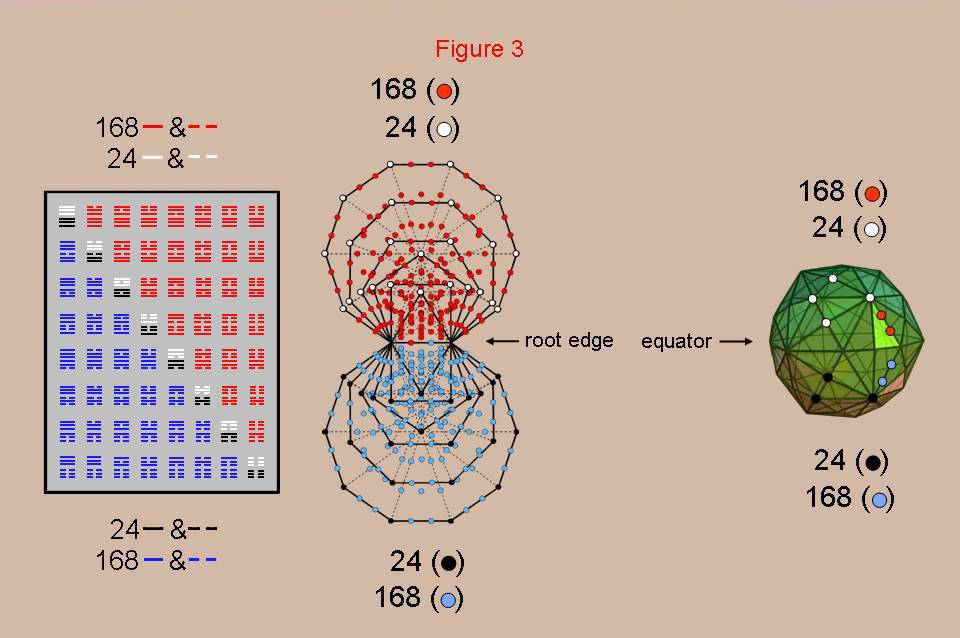Isomorphism between the I Ching, the inner           Tree of Life & the disdyakis triacontahedron. 7

Figure 4

The outermost polygon in the inner Tree of Life is the dodecagon. 168 extra yods are needed (84 in six sectors) to construct each of its 12 sectors from three tetractyses. The disdyakis triacontahedron has 84 edges and 24 vertices and 60 triangles above the XY plane, that is, another 84 geometrical elements. Similarly below this plane, which contains 12 vertices and 12 edges. Hence, the 84:84 division of yods in the dodecagon has its counterpart in the 84:84 division of geometrical elements above or below the equator of the disdyakis triacontahedron.

The centres of the two dodecagons correspond to the A vertices at the top and bottom of the polyhedron. The 12 corners of each dodecagon correspond to the 12 vertices & edges in the XY plane and their mirror images. The two sets of 84 new yods in each dodecagon correspond to the 84 edges and 84 vertices/triangles above or below the XY plane. Each yod in the pair of dodecagons symbolizes a geometrical element composing the disdyakis triacontahedron. The yods denote the ‘bits of information’ needed to construct the polyhedral form of the inner Tree of Life.

8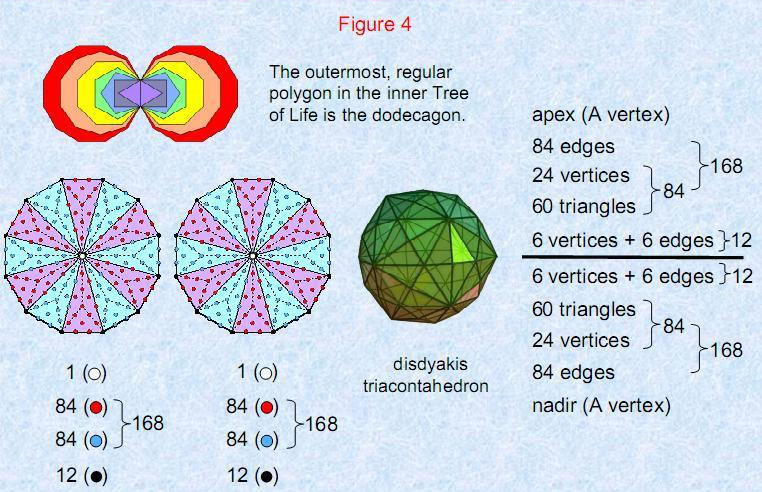Isomorphism between the disdyakis triacontahedron and                        the pair of dodecagons of the inner Tree of Life. 9

Figure 5

Each of the 120 triangular faces of the disdyakis triacontahedron has an A, B & C vertex. The polyhedron has 60 AB edges, 60 BC edges and 60 AC edges. The 12-sided polygon in the equator has four AB edges, four BC edges and four AC edges. This leaves 56 sets of three different types of edges above and below the XY plane, when the Z axis passes though two diametrically opposite A vertices, that is, 168 edges in 56 sets of three shape its 3-dimensional form.

Compare that property of the disdyakis triacontahedron with the 56 triangles with 168 vertices needed for the {3,7} tiling in the hyperbolic plane of the 168 symmetries of the Klein quartic belonging to PSL(2,7). Also notice that there are 24 vertices, 84 edges and 60 triangles, that is, 168 geometrical elements, between the XY plane and the apex of the polyhedron and 168 elements between this central plane and its nadir. These (168+168=336) elements correspond to the 336 triangles needed in the {7,3) tiling of the 168 automorphisms and 168 anti-automorphisms of the Klein quartic. The two sets of 168 symmetries correspond to the 168 geometrical elements either above or below the equator of the disdyakis triacontahedron between opposite vertices. The PSL(2,7) and SL(2,7) groups are embodied in the geometry of this polyhedron. PSL(2,7) is isomorphic to SL(3,2), the symmetry group of order 168 of the Fano plane that represents the algebra of the octonions, which form a natural representation of the Lie algebra of E8, the unified superstring gauge symmetry group. This indicates that a connection exists between the geometry of the disdyakis triacontahedron and superstring dynamics.

10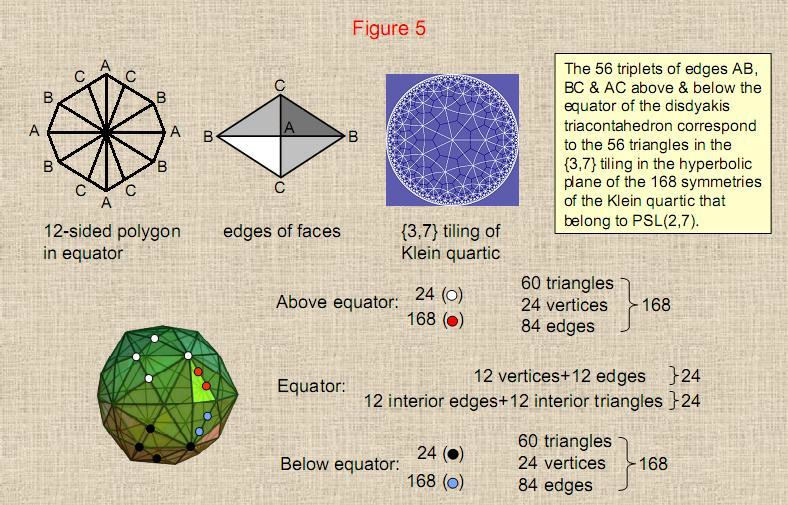The disdyakis triacontahedron is the polyhedral embodiment of SL(2,7). 11

Figure 6

The I Ching table, the Sri Yantra, the inner Tree of Life, the Klein configuration and the disdyakis triacontahedron exhibit a universal 168:168 pattern in their geometries. It is highly implausible that this is a coincidence. Instead, it strongly indicates the relevance to theoretical physics (in particular, to string/brane theory) of the group SL(2,7) of order 336, which is the double-cover group of PSL(2,7) of order 168. It is further suggested by the fact that the basic constituent of matter described by Annie Besant and C.W. Leadbeater over a century ago consists of ten closed curves, each of which is a circularly polarized standing wave that makes 168 oscillations in half a revolution around the spin axis of the particle. Its inner and outer halves with 168 helical turns per half-revolution are the manifestation of the mirror/inversion symmetry of these isomorphic objects of sacred geometry or – in the case of the I Ching table – the Yin/Yang balance of the 168 unbroken lines and the 168 broken lines of its 64 hexagrams.

12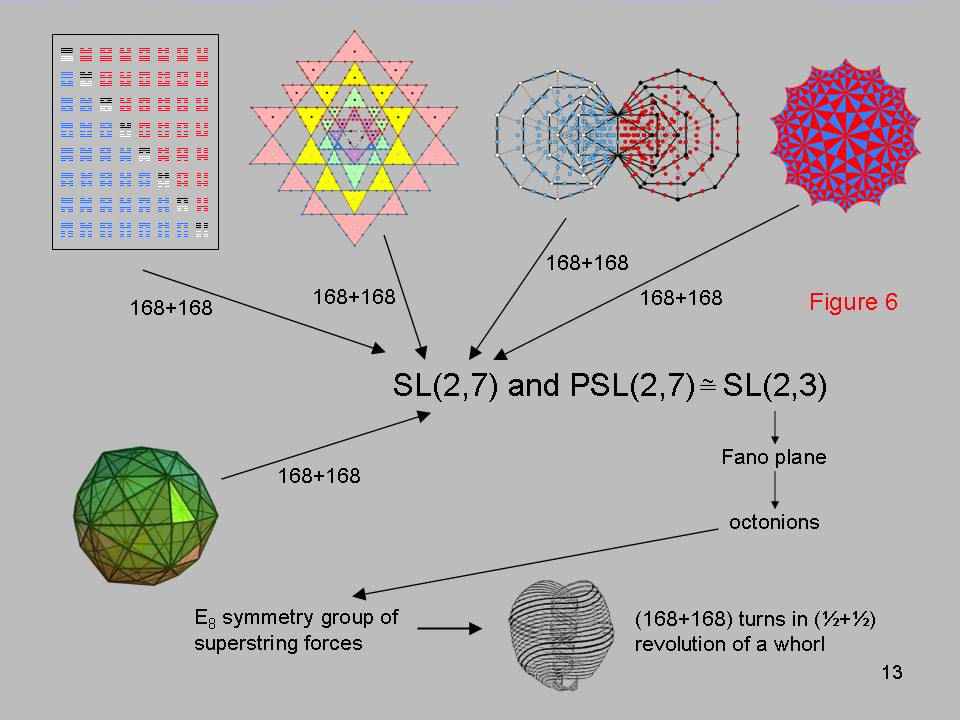Figure 7

Each Sephirah has a corresponding Godname, Archangel, Angelic Order & Mundane Chakra. The Mundane Chakra of Malkuth, the last Sephirah of the Tree of Life signifying the physical universe, is called in Hebrew “Cholem Yesodeth.” It means “breaker of the foundations.” Its gematria number value is 168. The number value of Cholem is 78 and the number value of Yesodeth is 90.

14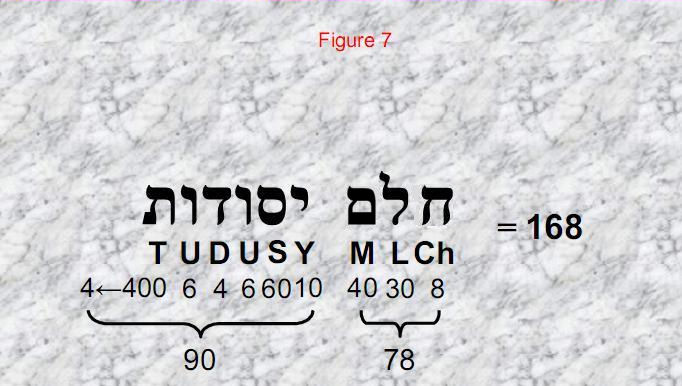Cholem Yesodeth, the Hebrew name of the Mundane Chakra of Malkuth                             (physical universe) has the gematria number value of 168.                       15

Figure 8

Consider the disdyakis triacontahedron constructed internally as well as externally from triangles. Each of its 180 edges is then the base of a triangle one of whose vertices is the centre of the polyhedron. Then suppose that these internal triangles are each divided into three triangles. This generates 168 vertices above and below the equator of the polyhedron with ‘A’ vertices (peaks of its 30 rhombic pyramids) at its apex and nadir. Surrounding the vertical axis joining these two vertices, there are 168 vertices & triangles above the equator, 168 vertices & triangles below the equator and 168 edges above and below it that are in its 120 faces. Surrounding the axis of the disdyakis triacontahedron are 660 triangles with 240 vertices, i.e., 900 geometrical elements, and 780 edges, making a total of 1680 geometrical elements. The split: 1680 = 780 + 900 matches (apart from the tetractys/Tree of Life factor of 10) the number values of the Hebrew words “cholem” and “yesodeth” making up the Mundane Chakra of Malkuth. Moreover, there are 1680 turns in each helical whorl of the basic unit of matter described by Besant & Leadbeater over a century ago and now identified as the E8×E8 heterotic superstring! This extraordinary embodiment of the superstring structural parameter 1680 in the geometry of the disdyakis triacontahedron is very strong evidence that it is the cosmic blueprint defining both the dynamics and structure of superstrings. Further confirmation that this is not a coincidence is the fact that there are 900 geometrical elements in the polyhedron down to the equator and 780 elements below it. The two words “yesodeth” and “cholem” define the numbers of geometrical elements making up, respectively, half the polyhedron and the remainder of it.

16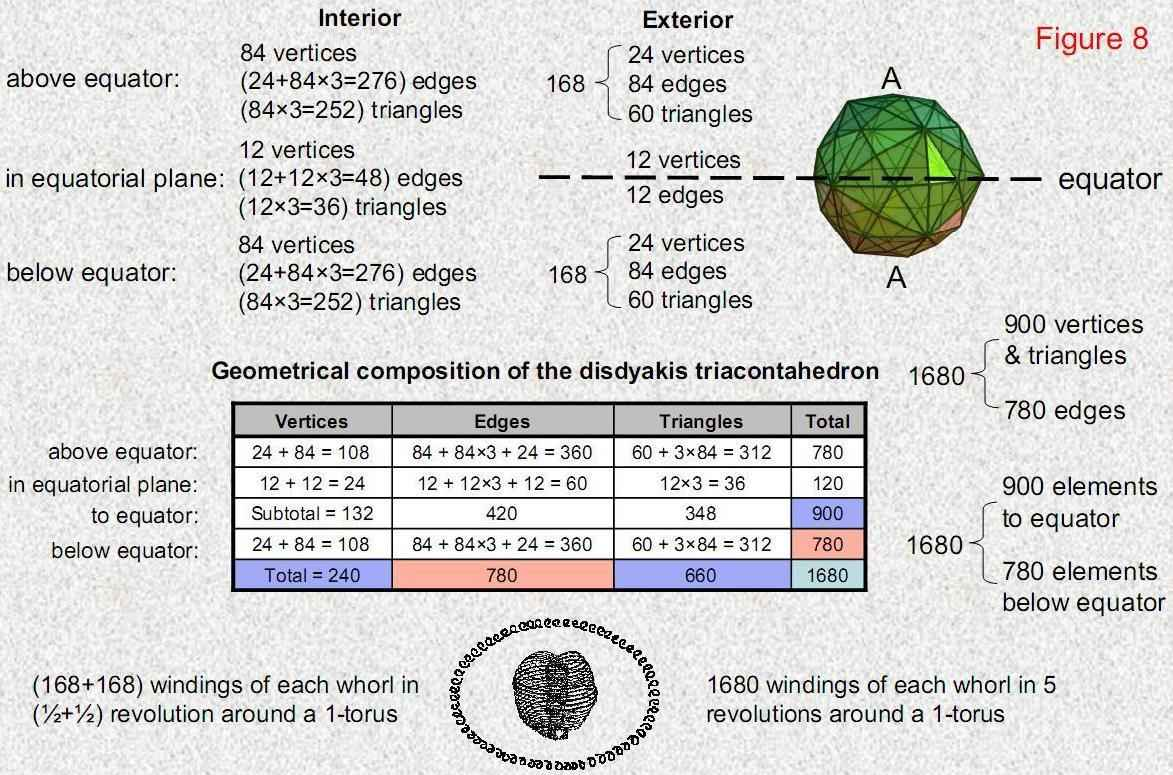Number values of Yesodeth (90) & Cholem (78) define the numbers of geometrical elements                    in the disdyakis triacontahedron to, respectively, its equator and below its equator. 17

Figure 9

The gematria number value 168 of Cholem Yesodeth is the number of yods other than corners associated with each set of the first six enfolded polygons making up the inner Tree of Life. The pentagon, hexagon & octagon have 90 yods and therefore embody the number value of Yesodeth. The triangle, square & decagon have 78 yods, so that they embody the number value of Cholem. The polyhedral counterpart of this is the triakis tetrahedron. When the interior triangles formed by its centre and edges are each divided into three sectors, there are 90 vertices & triangles surrounding an axis passing through two opposite vertices and 78 edges. As the Catalan solid with the fewest number of edges (see the table on p. 9 in Part 2 of Article 40), it is the only semi-regular polyhedron with this property. The Catalan solid with the largest number of edges is the disdyakis triacontahedron. Similarly constructed, it has 900 vertices & triangles and 780 edges surrounding an axis joining two opposite vertices, that is, exactly ten times the corresponding numbers for the triakis tetrahedron. It is as if the disdyakis triacontahedron is the tetractys of the world of polyhedra and the triakis tetrahedron is one of its ten yods.

This hidden, ten-fold nature of the disdyakis triacontahedron is confirmed by the fact that, associated with the 60 polygons of the first six types enfolded in ten overlapping Trees of Life are 900 yods other than corners in the ten pentagons, ten hexagons and ten octagons and 780 yods other than corners in the ten triangles, ten squares & ten decagons (“associated” means that only one of the two hexagonal yods in each shared, root edge is included in the count, the other hexagonal yod being associated with the other set of polygons).

18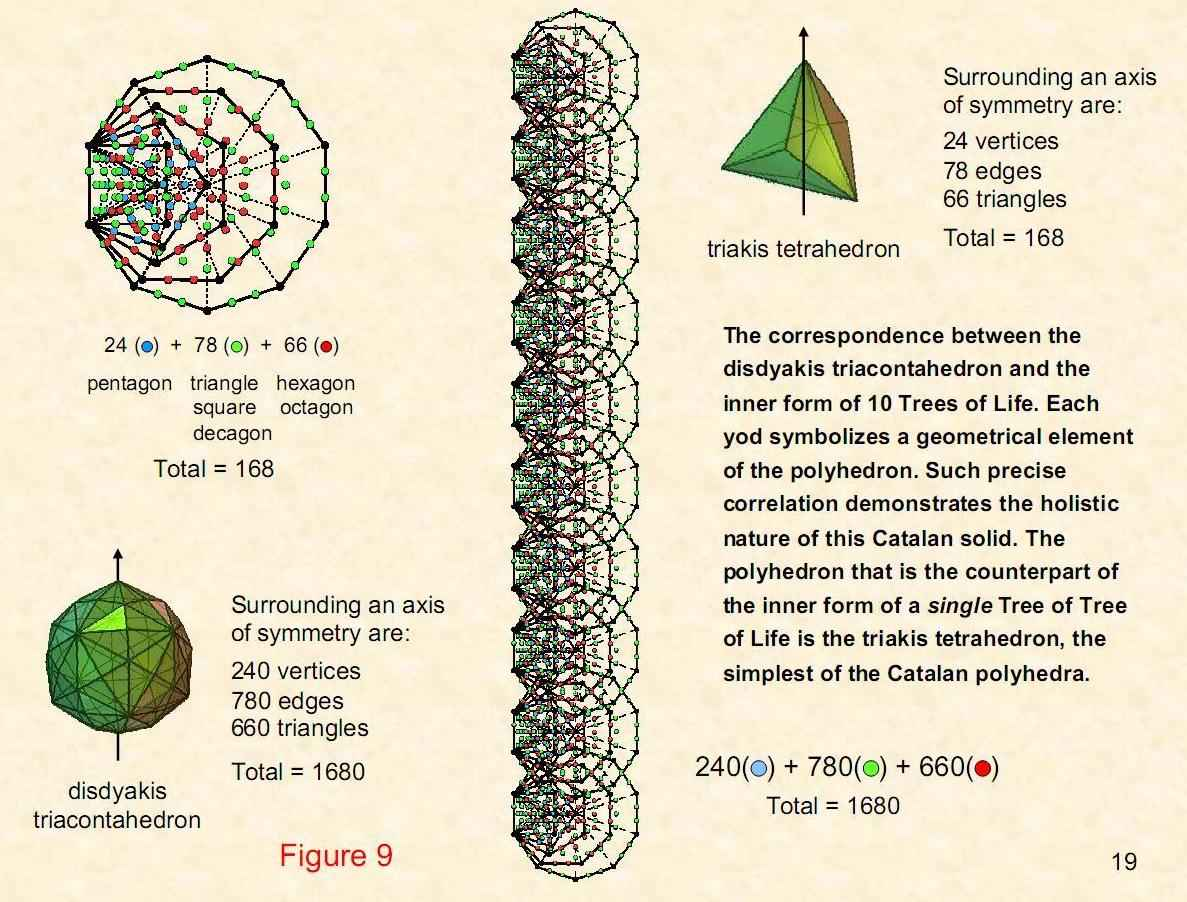Figure 10

Above or below the equator of the disdyakis triacontahedron between two opposite vertices are 168 geometrical elements. They comprise 4 edges & 60 triangles, i.e., 144 elements, and 24 vertices. This 24:144 division of the geometrical parameter 168 is found in the {3,7} mapping onto a 3-torus of the 168 automorphisms of the Klein quartic, as now explained: the 3-torus is topologically equivalent to a tetrahedral array of four triangular prisms to whose 12 square faces is attached the 12 square faces of six antiprisms (see pp. 12 & 13 of Part 3 of Article 40) The four triangular prisms have eight triangles with 24 vertices; the six square antiprisms have 48 triangles with 144 vertices. So 56 triangles with (24+144=168) vertices tile the 3-torus. The 24 vertices of the triangular prisms correspond to the 24 vertices above or below the equator of the disdyakis triacontahedron. The 144 vertices of the square antiprisms correspond to the 144 edges & triangles above or below the equator. The two halves of the polyhedron outside the equator correspond to the 3-torus mapping the 168 automorphisms of the Klein quartic and its turned inside-out version, which maps its 168 anti-automorphisms.

This correlation is further evidence that the disdyakis triacontahedron embodies the symmetry of SL(2,7) displayed by the Klein quartic.

20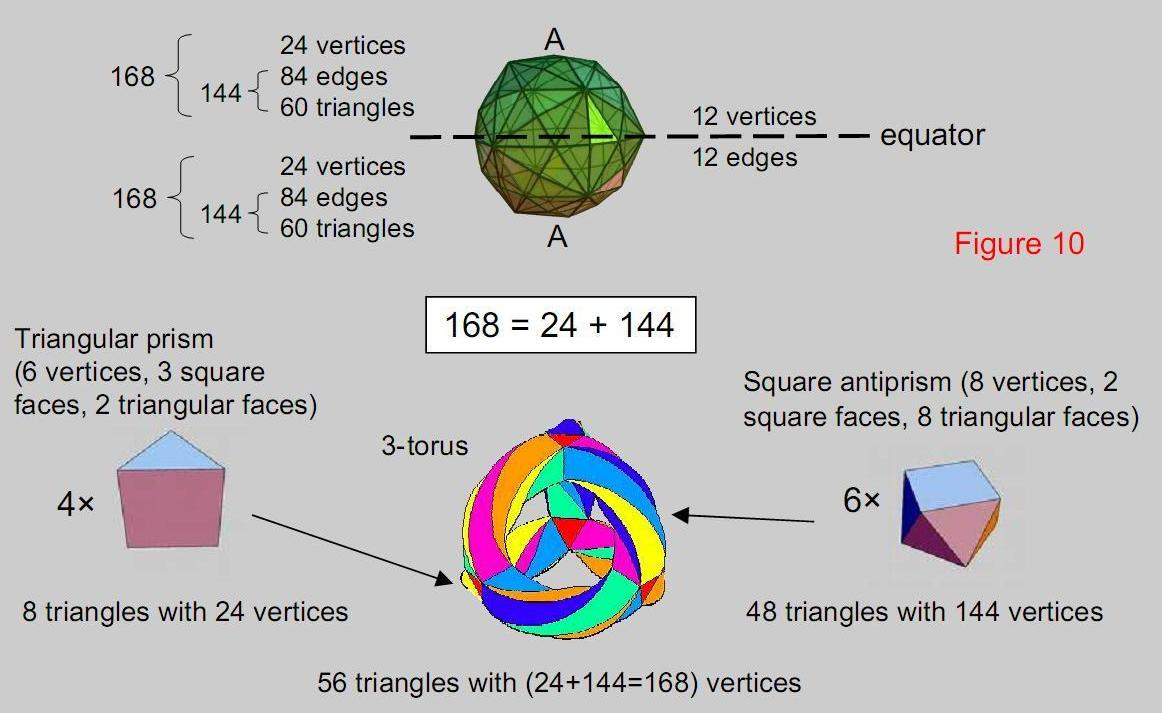Isomorphism between the (24+144) geometrical elements of the disdyakis triacontahedron above & below the  equator and the (24+144) vertices of the (8+48) triangles tiling the 3-torus and its turned inside-out form 21

Figure 11

The same 24:144 division is displayed by the yods in the set of the first six enfolded polygons of the inner Tree of Life. The 168 yods other than corners associated with each set includes 24 that belong to the pentagon. So each of these 24 yods symbolize a vertex of the eight triangles in the four triangular prisms joining the six square antiprisms, whilst the 144 yods in the five other polygons denote the 144 vertices of the 48 triangles in the square antiprisms. As these vertices denote automorphisms of PSL(2,7), one of which is the identity element, it is tempting to identify the single hexagonal yod in the root edge that is associated with one set of polygons as the identity I and the other hexagonal yod associated with the other set as -I. The two identical sets of six polygons, each with 168 yods other than corners, correspond to the {3,7} tiling of the 3-torus by 56 triangles with 168 vertices mapping the 168 automorphisms and by the 56 triangles on its turned inside-out version mapping the 168 anti-automorphisms. Every yod in the six enfolded polygons that is not one of its corners symbolizes a symmetry of the Klein quartic. The two mirror-image sets of polygons correspond to the 3-torus and to a 3-torus turned inside out.

22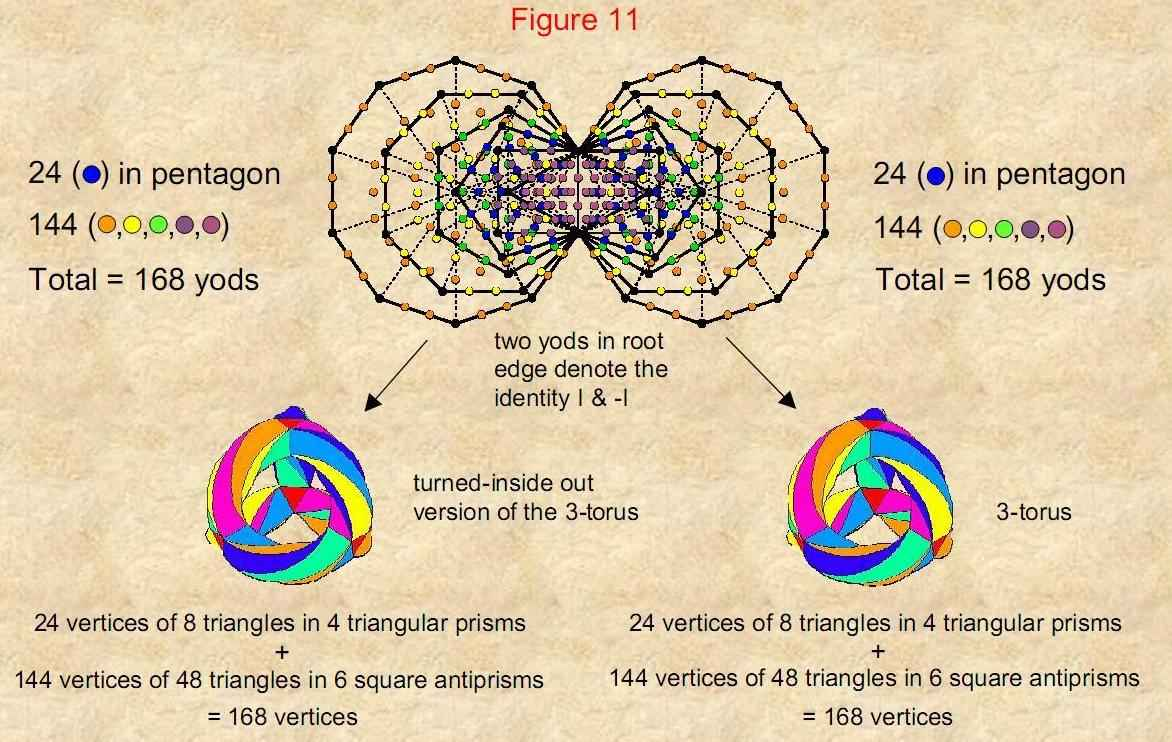Each of the two sets of the first 6 enfolded polygons of the inner Tree of Life has (24+144) yods. One                            set is isomorphic to the (24+144) vertices of the 56 triangles in the {3,7} tiling on a 3-torus of the 168                            automorphisms of the Klein quartic. The other mirror-image set is isomorphic to the (24+144) vertices                            of the 56 triangles on the turned inside-out 3-torus tiling the 168 anti-automorphisms. 23

Figure 12

As further confirmation of the remarkable isomorphism between the inner Tree of Life, the disdyakis triacontahedron and the tiling of the 168 symmetries of the Klein quartic onto a 3-torus, the same 24:144 division of the respective yods, geometrical elements and vertices of triangles is exhibited in the boundaries of the two sets of the first six polygons. 168 yods lie on their edges outside their shared root edge. Of these, 24 red yods are corners of the pair of hexagons and decagons outside their shared root edge. 144 black yods lie on the 60 external edges of the other eight polygons. The Godname EL of Chesed with number value 31 prescribes the first six enfolded polygons because they have 31 sides. The Godname YAH with number value 15 prescribes the enfolded hexagon and decagon with 15 edges.

24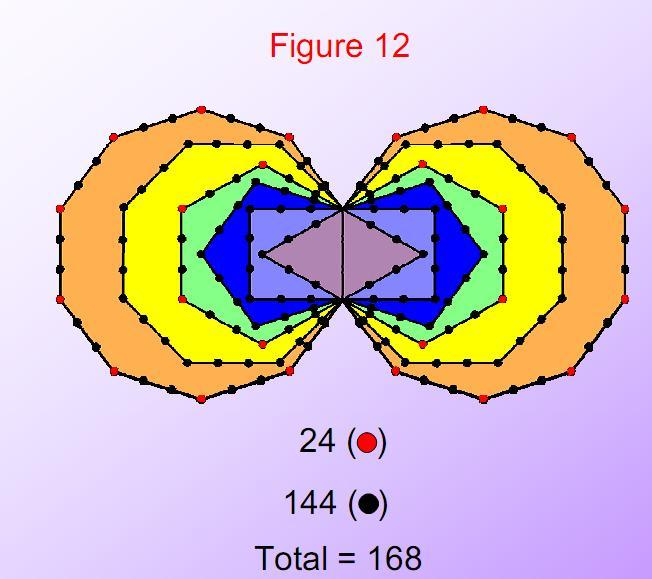The pair of 24:144 divisions of: 1. geometrical elements in the disdyakis triacontahedron, 2. yods in the first (6+6) regular polygons of the inner Tree of Life and 3. triangles in the {3,7} tiling of the 168 automorphisms and the 168 antiautomorphisms of the Klein quartic on a 3-torus is a fundamental property of holistic systems. This basic division appears not only in each set of 6 enfolded polygons but also in the pair of them. There are 24 corners of the two hexagons and the two decagons outside the shared root edge and 144 yods on the external edges of the two sets of polygons. In other words, 168 yods are needed to delineate their shapes, confirming the character of this number as a structural parameter of the heterotic superstring as the microscopic manifestation of the Tree of Life blueprint.   25

Figure 13

Each of the ten closed curves of the basic unit of matter described by Annie Besant and C.W. Leadbeater is a helix with 1680 turns. As each curve winds five times around the spin axis of the particle, it makes 336 helical turns in one revolution, that is, 168 turns in a half-revolution. This 168:168 division corresponds to:

1. the (168+168) geometrical elements in the faces of the disdyakis triacontahedron above and below its equator;

2. to the 168 automorphisms and 168 anti-automorphisms of the Klein quartic as represented by the 168 vertices of the 56 triangles tiled on the 3-torus and the 168 vertices of the 56 triangles tiled on its turned inside-out version;

3. the (168+168) yods other than corners in the first (6+6) enfolded polygons;

4. the (168+168) lines/broken lines in the 56 off-diagonal hexagrams of the I Ching table.

26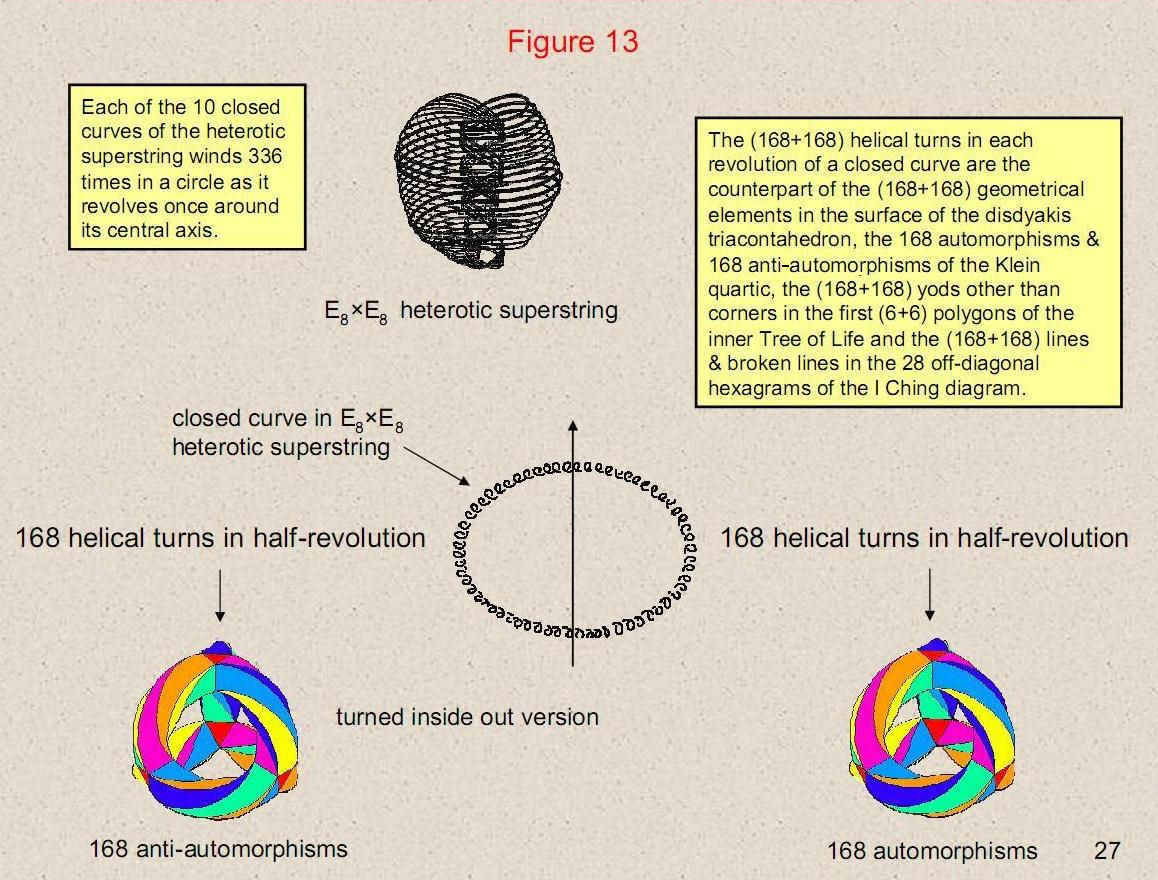Figure 14

When the 47 sectors of the seven enfolded polygons are transformed into 2nd-order tetractyses (the next order above the tetractys in which yods are replaced by tetractyses), they contain 3360 yods. This is the number of helical turns made by all ten closed curves of the E8×E8' heterotic superstring when they make one revolution around the spin axis of the spin-½ particle. Each yod denotes a turn. Physically, it is an oscillation of the circularly polarized standing wave running around the curve. The Godname ELOHIM with number value 50 prescribes the fundamental constituent of quarks because its ten whorls make 50 revolutions about its spin axis. Assigning this number to each of the yods in the inner Tree of Life generates the number 16800 as the number of circularly polarized oscillations in the ten closed curves of the heterotic superstring.

28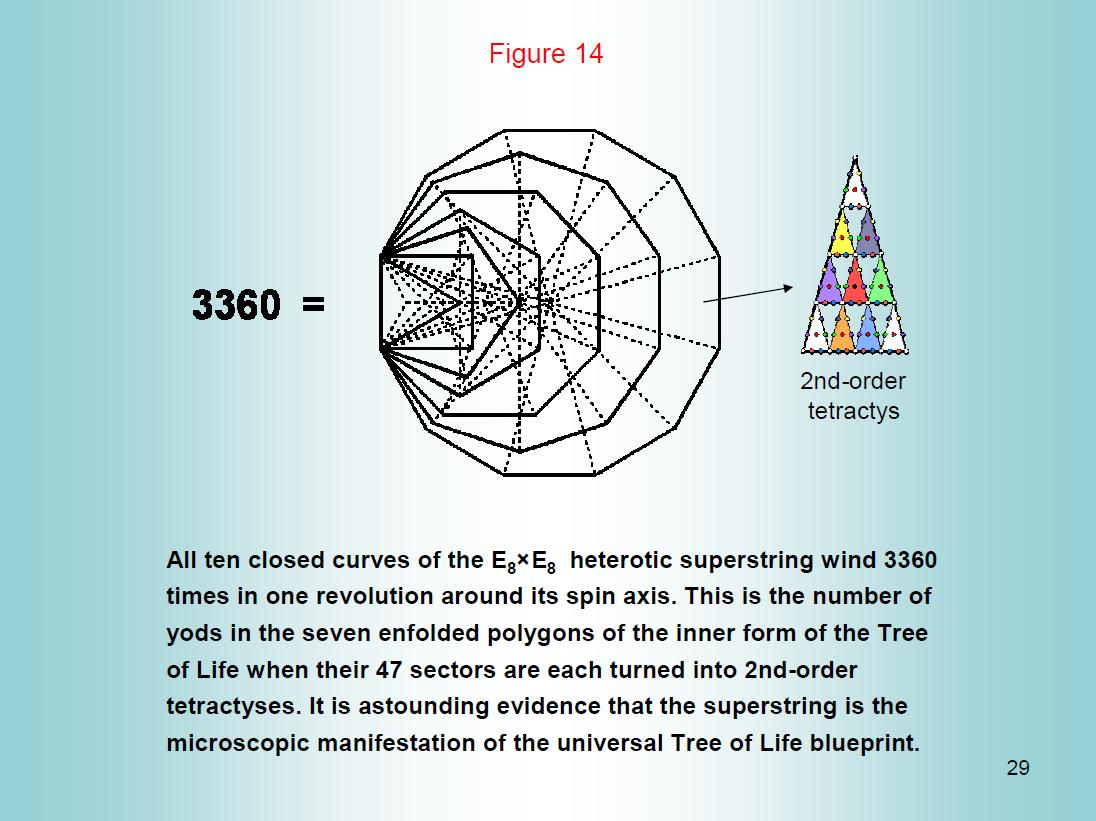Figure 15

Assigning the number 10 (the Pythagorean Decad) to the 336 yods on the 126 edges of the 42 triangles of the 3-dimensional Sri Yantra generates the number 3360. This demonstrates how the Sri Yantra and the inner Tree of Life are equivalent representations of holistic systems like the heterotic superstring. Each half of the Sri Yantra with 168 yods defines the number (1680) of circularly polarized oscillations made by all ten closed curves of the superstring during half of a revolution around its spin axis. The inner and outer halves of the superstring correspond to the two halves of the Sri Yantra. They are prescribed by the Godname EHYEH with number value 21 because each half is made of 21 triangles.

30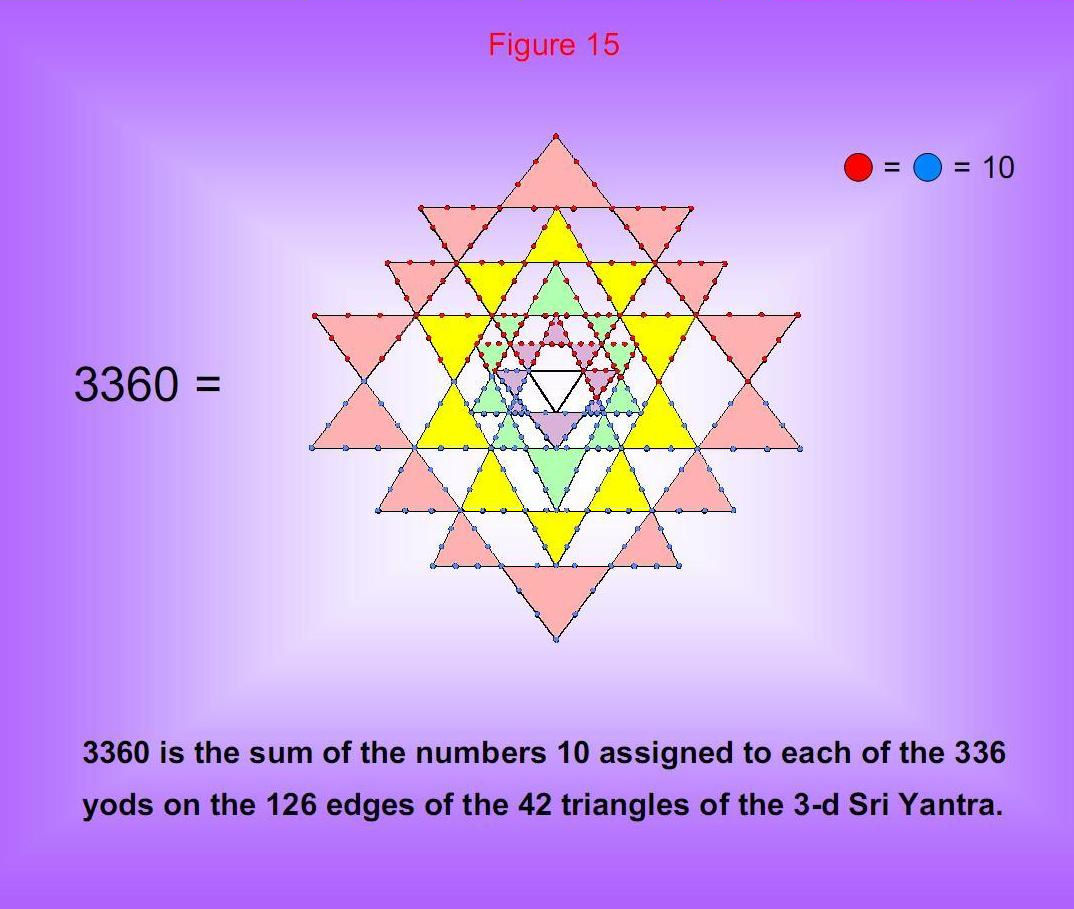31

Figure 16

The Godname of Malkuth is ADONAI. Its number value is 65. It prescribes the lowest ten overlapping Trees of Life because they have 65 Sephiroth. When their triangles are each constructed from three tetractyses, there are 1680 yods below the top of the tenth tree – the 65th Sephirah. Each yod symbolizes a circularly polarized oscillation in each closed curve of the heterotic superstring. It shows how ADONAI, the Godname of the Sephirah signifying the physical universe, prescribes the form of the basic unit of matter.

There are 1680 yods outside the shared edges that lie on the edges of the first six polygons enfolded in each of ten overlapping Trees of Life on either side of the central Pillar of Equilibrium. This demonstrates the shape-defining character of this structural parameter of the E8×E8' heterotic superstring.

32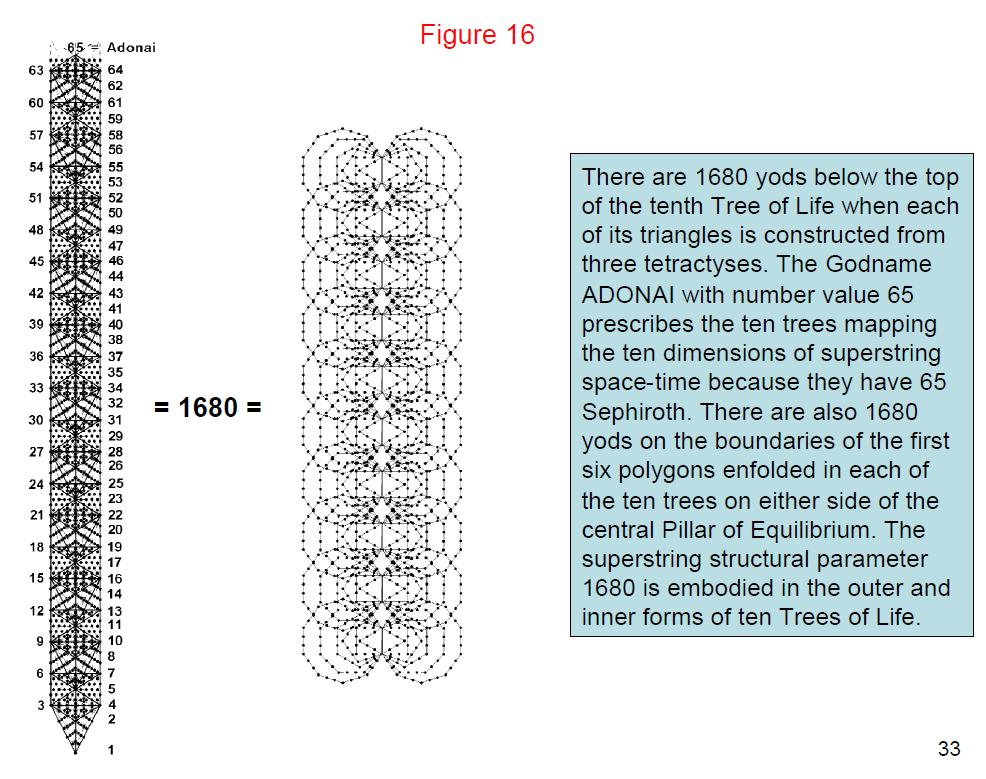Figure 17

Suppose that the 50 faces of the five Platonic solids are constructed from tetractyses. The four faces of the tetrahedron have 48 hexagonal yods, the eight faces of the octahedron have 96 hexagonal yods, the six faces of the cube have 96 hexagonal yods, the 20 faces of the icosahedron have 240 hexagonal yods and the 12 faces of the dodecahedron have 240 hexagonal yods.

34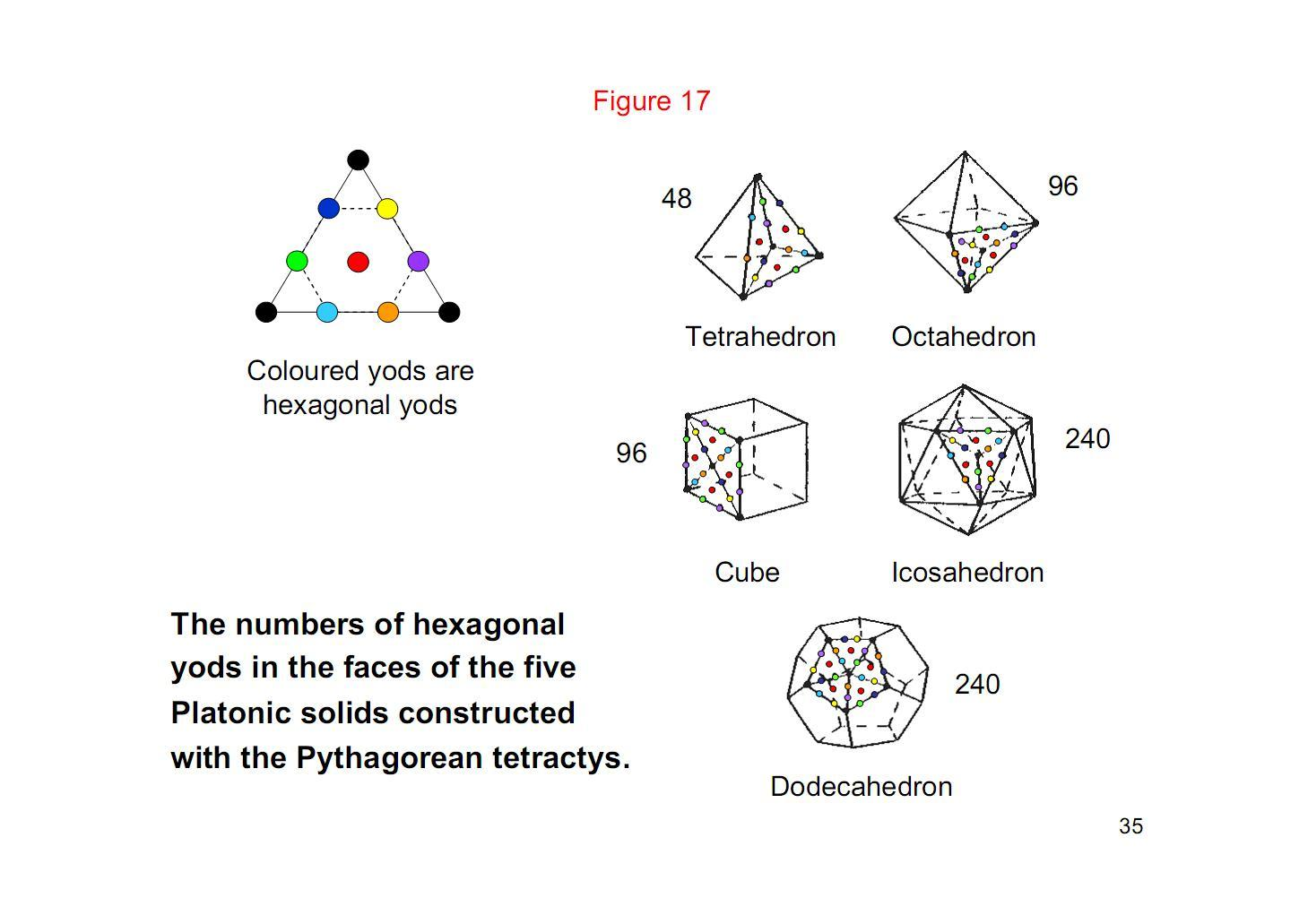Figure 18

The 62 vertices of the disdyakis triacontahedron generate 10 tetrahedra, five octahedra, five cubes, one icosahedron, one dodecahedron, five rhombic dodecahedra and one rhombic triacontahedron. The 28 polyhedra contain 3360 hexagonal yods when their faces are constructed from tetractyses. The superstring structural parameter 3360 is embodied in the polyhedral potential of the disdyakis triacontahedron, demonstrating its remarkable archetypal character. Notice that the four Platonic solids representing the four elements of Earth, Water, Air and Fire have 1680 hexagonal yods. They comprise 1440 hexagonal yods in the solids representing Fire, Air & Earth and 240 hexagonal yods in the icosahedron representing Water. The same 240:1440 division is exhibited in the other seven polyhedra. It is another manifestation of the division discussed on pages 20-25 in the contexts of the disdyakis triacontahedron, the tiling on the 3-torus of the 168 symmetries of the Klein quartic and the yods in the first six enfolded polygons.

36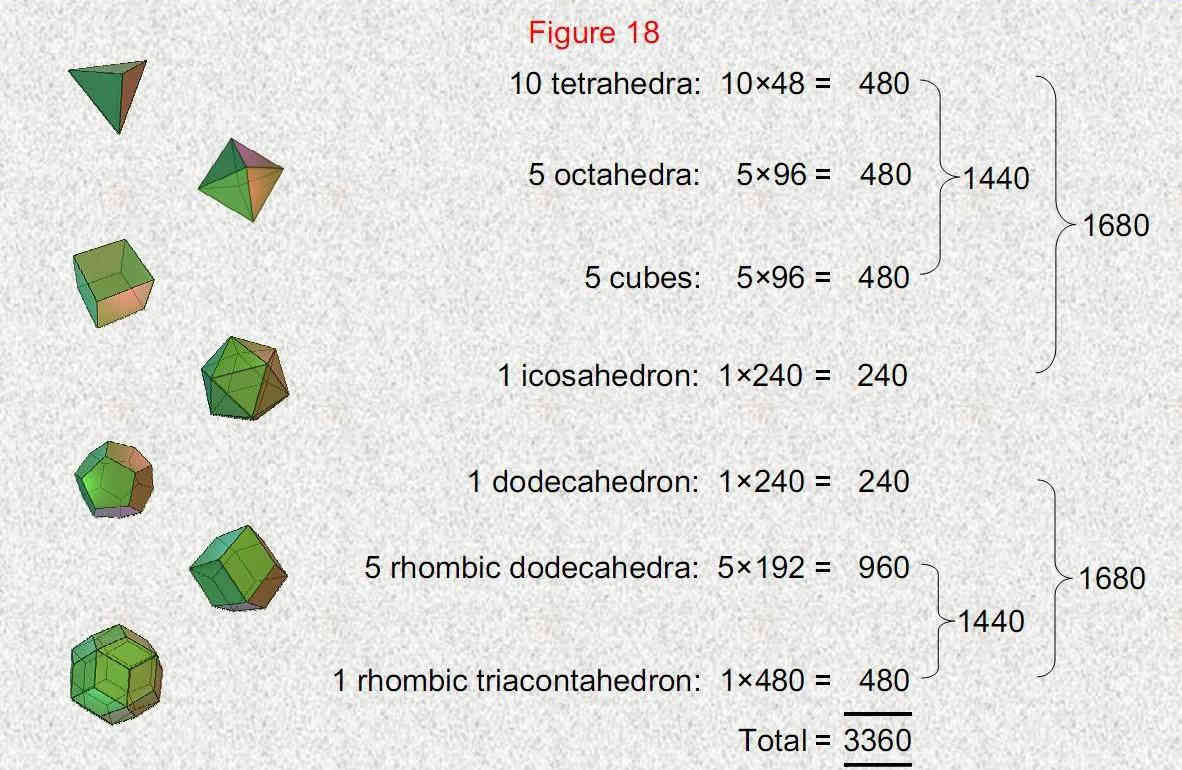3360 hexagonal yods are needed to construct from tetractyses the 28 regular and                               semiregular  polyhedra generated by the vertices of the disdyakis triacontahedron 37

Figure 19

A Hypothesis

Hypothesize that each of the three generations of basic fermions has seven colour states, one defining leptons and six defining subquarks. Each generation consists of two weak isospin states, each isospin state consists of two supersymmetric states, each supersymmetric state is a particle and its antimatter counterpart and each particle exists in left-hand and right-hand parity states. This implies that there are 336 different particles made up of 168 left-handed states and 168 right-handed states. This would correspond to the 168 geometrical elements in each half of the disdyakis triacontahedron between opposite vertices and the equator. The inversion symmetry of the polyhedron would naturally correspond to the distinction between left-handed particles and their mirror image right-handed counterparts. Moreover, the 24 left-handed leptons would correspond to the 24 vertices above the equator, the 24 right-handed leptons would correspond to the 24 vertices below the equator and the 144 edges & triangles in each half of the polyhedron would correspond to the 144 left-handed and 144 right-handed subquarks. The 24 triangles in each of the seven segments of the {7,3} tiling of the Klein quartic would signify the 24 left-handed or right-handed particles in each of the seven colour states.

As we have seen (pp. 20-25), the inner Tree of Life, the I Ching table, the Sri Yantra and the disdyakis triacontahedron also embody the number 168 and its division into 24 and 144. The physical meaning of this would be that the former is the number of leptons of a given handedness (3×2×2×2) and that the latter is the number of subquarks of the same handedness (3×6×2×2×2). Do, therefore, the 168 automorphisms and 168 anti- automorphisms of the Klein quartic have a physical interpretation in terms of these states of matter fields? Is the field of order 7 in PSL(2,7) the seven colour states?

38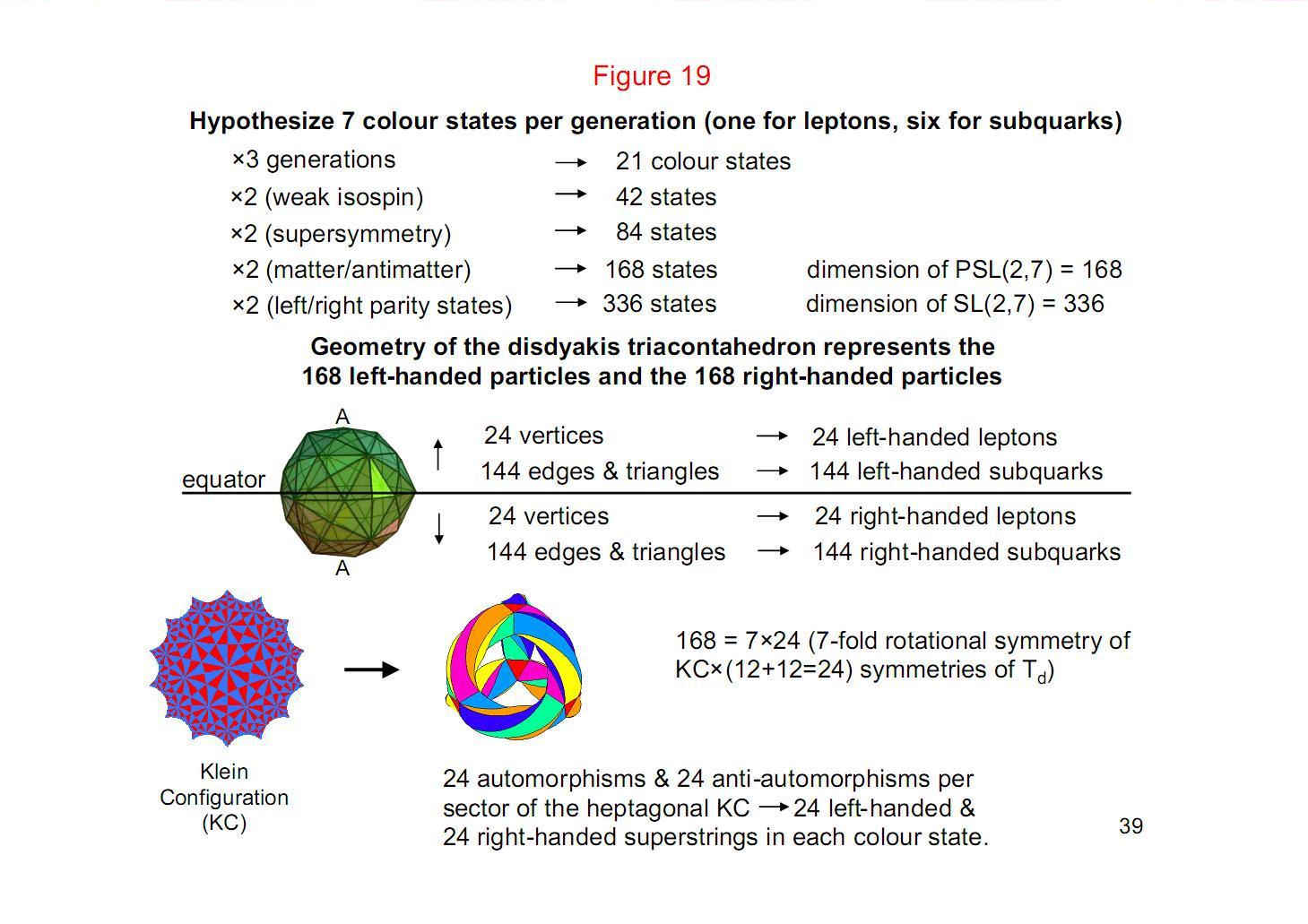Figure 20

It requires seven colours to colour a map drawn on a 2-torus. K7, the complete graph of order 7, can be embedded on a 2-torus. This is the topology of each whorl of the unit of matter described by Besant & Leadbeater. The seven vertices of K7 could symbolize the seven colour states of each generation of superstring, the 21 straight lines joining its vertices corresponding to the 21 colour states of the three generations of superstrings. Turning the straight lines into bi-directional arrows generates (7+7=14) arrows pointing along the edges of the graph and (14+14=28) arrows pointing inwards, that is, 42 arrows. This correlates with the 42 triangles of the Sri Yantra, the 14 arrows corresponding to the 14 triangles in the fourth layer and the 28 arrows corresponding to the 28 triangles in the first three layers. It would seem that the correlation, if not accidental, means that the Sri Yantra is defining the seven-fold nature of holistic systems, e.g., the musical intervals above the tonic, the seven musical scales, the seven unit imaginary octonions and the seven colour states of superstrings.

40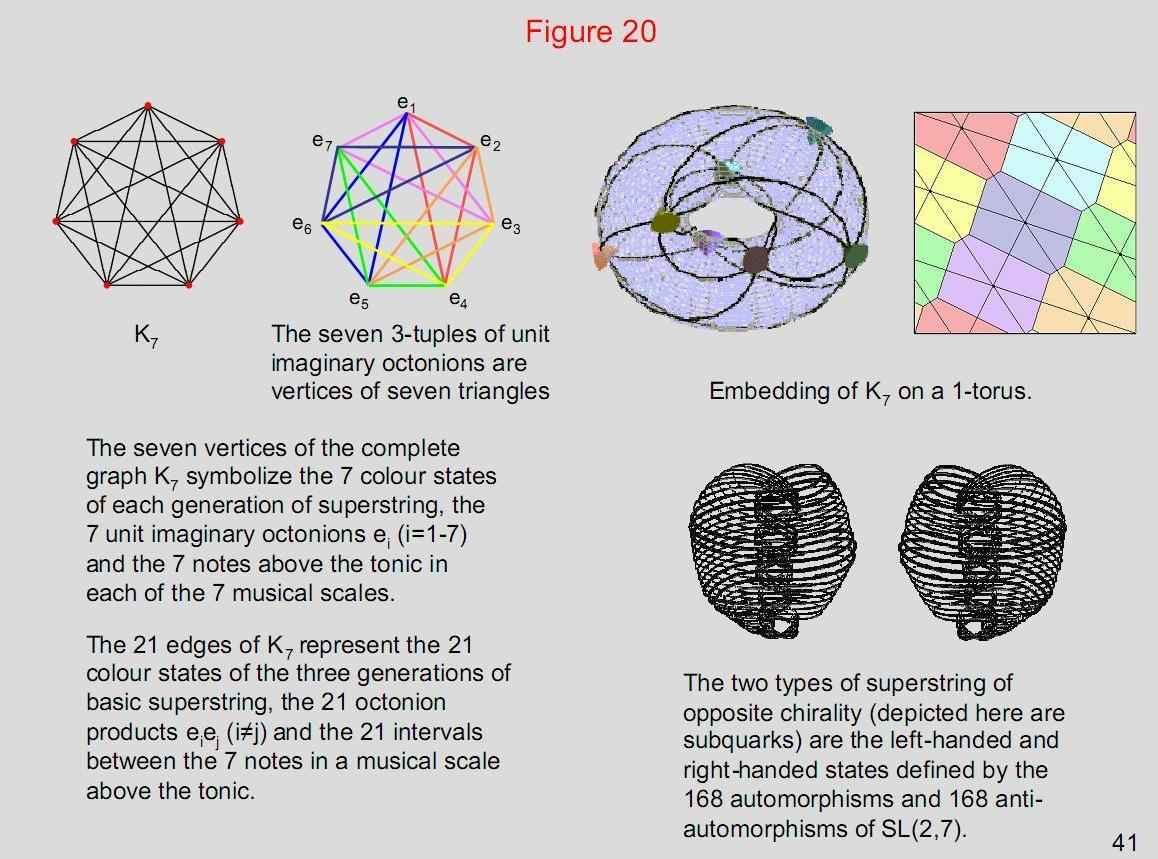Figure 21

The 168 vertices of the 24 heptagons in the {7,3} mapping of the 168 automorphisms of the Klein quartic may denote the 168 particles in 24 sets of seven colour states. The 168 vertices of the 56 triangles in the {3,7} mapping of the 168 automorphisms may denote the 168 particles made up of three generations of 56 states.

42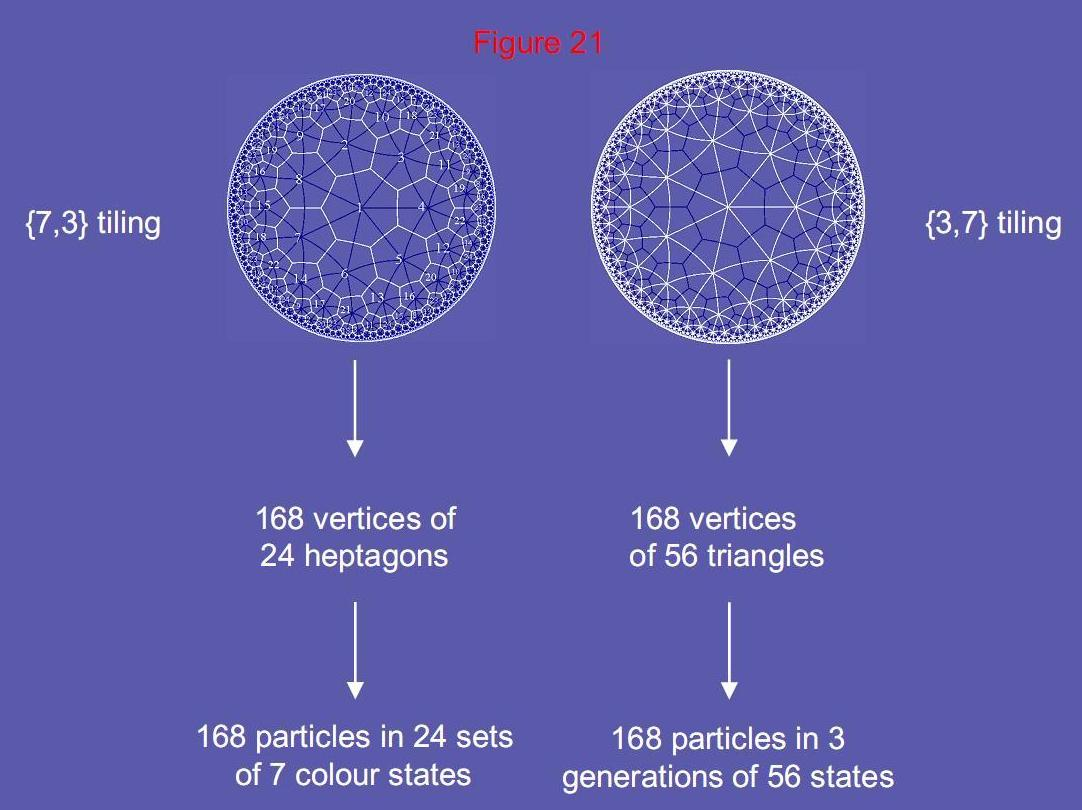A physical interpretation of the Klein configuration. 43

Figure 22

The first six enfolded polygons have 26 corners. One corner on the root edge is associated with one set of polygons and the other corner is associated with the other set. The topmost corner of the hexagon coincides with the lowest corner of the hexagon enfolded in the next higher tree. This means that the 42 polygons enfolded on each side of seven overlapping Trees of Life have 168 corners associated with them that are truly intrinsic to them because none belong to the polygons enfolded in the next higher tree. The number of independent degrees of freedom represented by these corners is therefore (168+168=336). They correspond in the {7,3} hyperbolic mapping of the 168 automorphisms and 168 anti-automorphisms of the Klein quartic to, respectively, the 168 red hyperbolic triangles and the 168 blue hyperbolic triangles. The counterpart of the 24 triangles in each of the seven segments of the Klein configuration is the set of 24 corners associated with each set of polygons. In each case, the 24 degrees of freedom may denote the 24 particles in each of the seven colour states. If this true, then the seven colour states are the manifestation of the seven Sephiroth of Construction. The colour state defining leptons would correspond to Malkuth and the six colour states defining subquarks would correspond to the two triads of Sephiroth of Construction above Malkuth.

44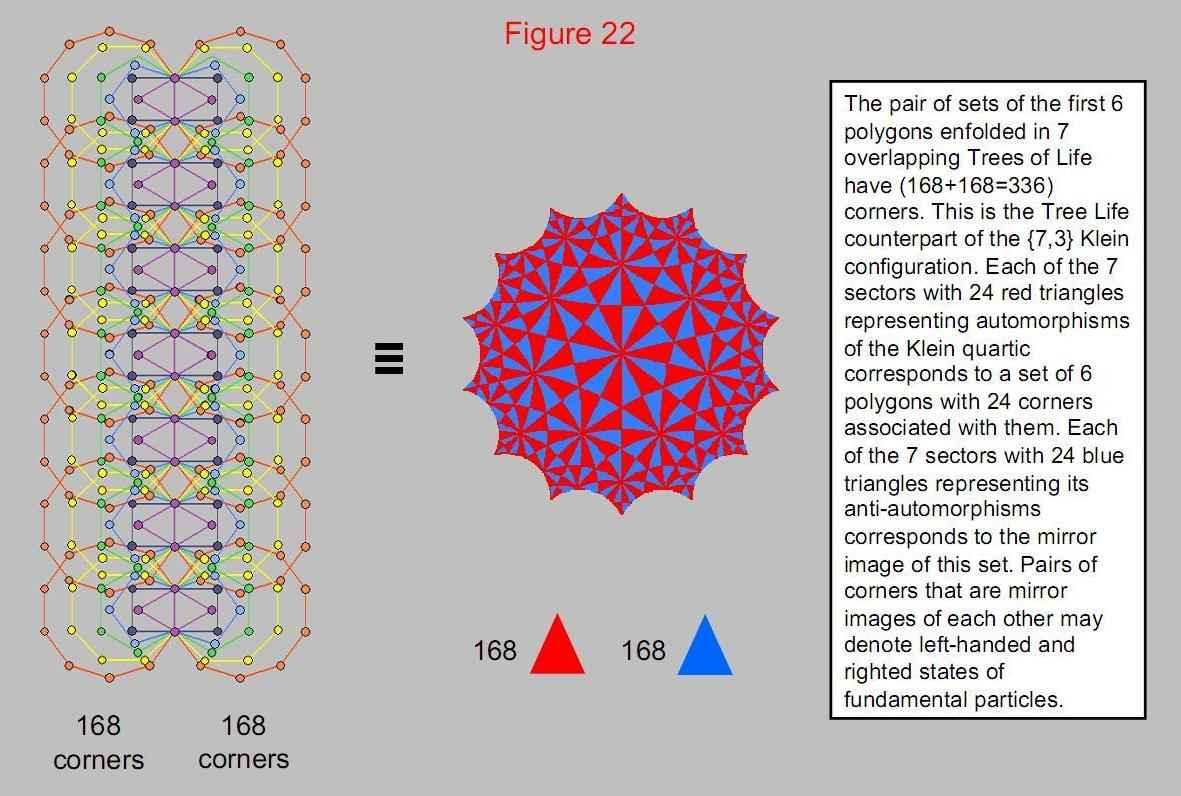The Tree of Life counterpart of the Klein configuration. 45

Figure 23

Earlier, we found that the I Ching table is isomorphic to the {3,7} mapping of the 168 automorphisms of the Klein quartic. The 56 trigrams of the 28 off-diagonal hexagrams in one half of the table correspond to the 56 hyperbolic triangles whose 168 vertices represent automorphisms. Just as there are 56 sets of three rows of lines/broken lines, so there are 56 particle states in each of three generations. The three rows of a trigram define the three possible generations of superstrings. The eight different trigrams denote the (23=8) states for each colour: weak isospin doublet(2)×supersymmetric doubling(2)×particle/antiparticle(2). The 168 lines and broken lines in the 28 hexagrams in one half of the table denote left-handed, superstring matter fields. The 168 lines and broken lines in the 28 hexagrams in the other half of the table denote right-handed particles.

Amongst the 90 intervals below the octave between the 14 types of notes found in the seven musical scales, there are 24 pairs of intervals and their complements. Three pairs of intervals are intervals between notes belonging to two different musical scales. They are not notes of these scales. This leaves 21 pairs of intervals that are notes of the seven scales. They correspond to the 21 basic, colour states of the three generations of superstrings and their antimatter, supersymmetric or weak isospin-doublet partners.

46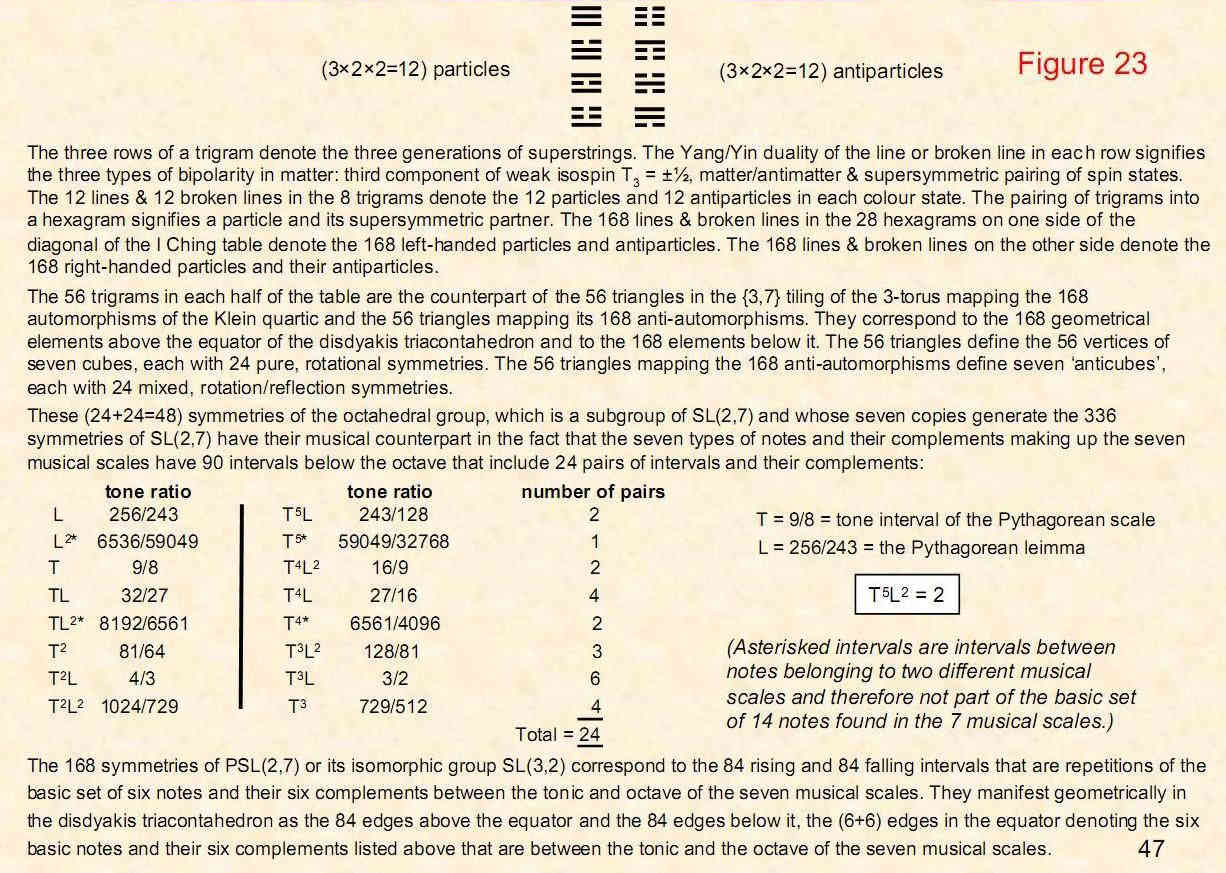Figure 24

The 24:168 division that, as we have seen, is displayed by various sacred geometries appears in the sequence of tone ratios of the notes of the Pythagorean scale. The perfect fifth of the fifth octave has the tone ratio 24. It is the tenth overtone and the 33rd note in the sequence. Indeed, every 33rd note increases in pitch by a factor of 24 and becomes the tenth overtone relative to the starting note. There are 24 overtones up to the note with tone ratio 192. So 14 overtones span a tone ratio difference of 168 between the note with tone ratio 24 and that with tone ratio 192. The notes of the seven musical scales have 189 intervals below the octave. They include 21 notes that are the second, third and fourth notes of each scale, leaving 168 intervals. Including the tonic, the octave and the unit interval between a note and itself, there are 192 intervals. They comprise 24 intervals (tonic, octave, unit interval and the 21 notes) and 168 intervals made up of the 21 complements of the 21 notes and 147 intervals between notes above the tonic (21 in each scale). Both the seven musical scales and the Pythagorean scale display the 24:168 division found for the inner Tree of Life, the I Ching table and the Sri Yantra.

48

Figure 24

Tone ratios of the notes in the Pythagorean musical scale

 C D E F G A B Number of overtones 1 1 9/8 81/64 4/3 3/2 27/16 243/128 0 2 2 9/4 81/32 8/3 3 27/8 243/64 2 3 4 9/2 81/16 16/3 6 27/4 243/32 4 4 8 9 81/8 32/3 12 27/2 243/16 7 5 16 18 81/4 64/3 24 27 243/8 11 6 32 36 81/2 128/3 48 54 243/4 15 7 64 72 81 256/3 96 108 243/2 20 8 128 144 162 512/3 192 216 243 26

Note G of the eighth octave (its perfect 5th) has the tone ratio 192. It is the 24th overtone. Note G of the fifth octave has the tone ratio 24. It is the tenth overtone. So 14 overtones span a tone ratio difference of 168 between the 24th overtone and the tenth overtone. Including the tonic, octave and unit interval common to the seven musical scales, there are 192 intervals between the notes of the seven scales. They comprise 24 intervals (tonic, octave, unit interval and the 2nd, 3rd & 4th notes of each scale) and 168 intervals. We have seen how the number 192 has appeared in various sacred geometries as a measure of a holistic system. For example, there are 192 yods associated with the first six enfolded polygons, of which 24 yods are their corners. There are 192 lines/broken lines in each half of the I Ching table, of which 24 lines/broken lines make up the eight basic trigrams, leaving 168 lines/broken lines in the 28 hexagrams above or below the diagonal. There are 192 yods in each half of the Sri Yantra (including the central triangle). They comprise the three hexagonal yods on the edges of the central triangle, the centres of the 21 triangles and the 168 yods on the edges of these triangles, i.e., they divided into a set of 24 yods and a set of 168 yods. Finally, there are 192 geometrical elements surrounding the centres of the seven separate, regular polygons. They comprise the 24 elements surrounding the centre of the hexagon and the 168 elements surrounding the centres of the other six polygons. The intervals between the notes of the seven musical scales have the same 24:168 division, demonstrating that they are elements of a holistic system.

49

Figure 25

42 triangles of the Sri Yantra surround the central one. Suppose that their 126 sectors are each constructed from three triangles that are then turned into tetractyses. Each triangle is composed of 46 yods. It comprises seven vertices, 15 edges and nine triangles, i.e., 31 geometrical elements, showing how the Godname EL of Chesed with number value 31 prescribes the most elementary shape when constructed from tetractyses. The value 1 of the Hebrew letter aleph (E) denotes the vertex at the centre of the triangle and the value 30 of the Hebrew letter lamed (L) denotes the 30 geometrical elements that surround it. As the nine triangles making up each triangle of the Sri Yantra have 15 edges, the Godname YAH of Chokmah with number value 15 prescribes these structural units, whilst the Godname EHYEH of Kether with number 21 prescribes them because there are 21 vertices and edges surrounding the centre of a triangle. 36 yods lying on edges of the nine tetractyses surround the centre, where 36 is the number value of ELOHA, Godname of Geburah. This is also the extra number of yods that have to be added to change the triangle from a tetractys with ten yods into one with 46 yods. Each triangle has 39 hexagonal yods and one centre (shown as black yods). There are (42×40=1680) of these yods in the Sri Yantra. Alternatively, there are 1680 yods other than outward tips of triangles and interior corners of triangles. They symbolize the 1680 helical turns in each closed curved of the heterotic superstring. As a geometrical paradigm of wholeness, the Sri Yantra represents the form of the basic unit of matter.

50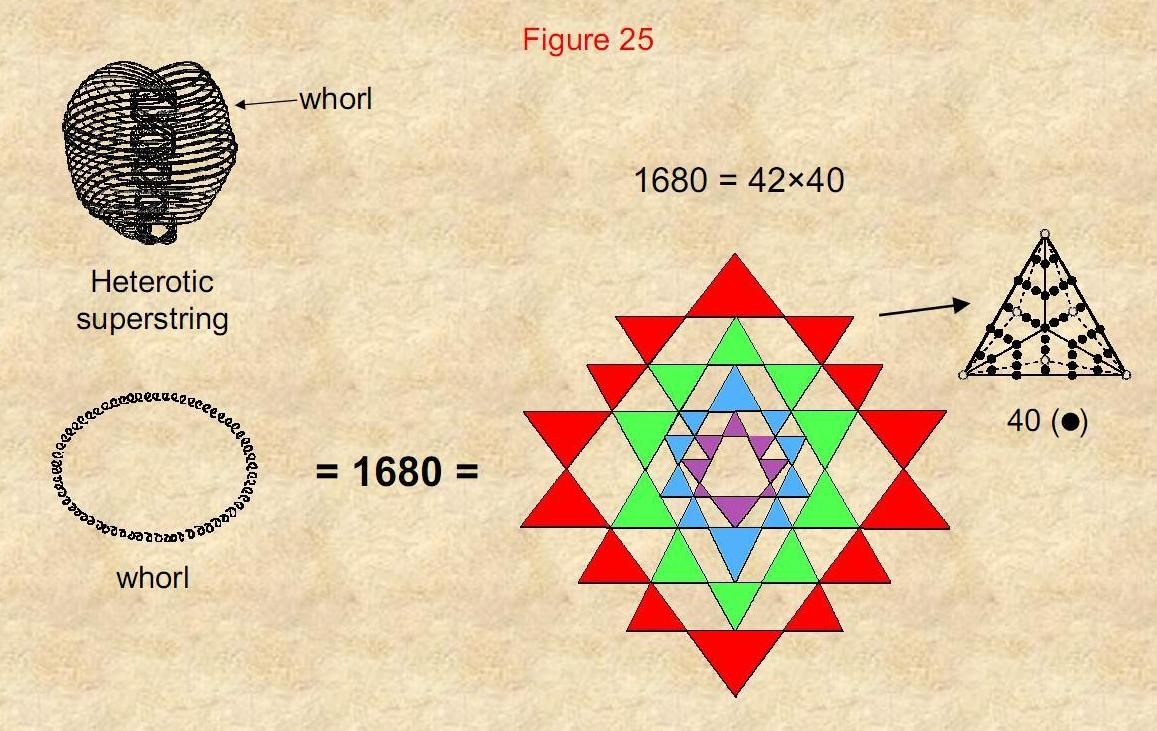The 1680 (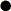) yods in the 42 triangles of the Sri Yantra symbolize                                the 1680 turns in each helical whorl of the heterotic superstring. 51

Home